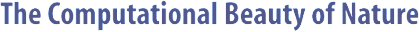Computer Explorations of Fractals, Chaos,Complex Systems, and Adaptation· title page · home* · cover artwork · jacket text · table of contents · the author* · ordering informationBook Contents
 · three themes · part synopses · selected excerpts · all figures from book · quotes from book · glossary from book · bibliography · slide showSource Code
 · overview &documentation · FAQ list* · download source code · java appletsMiscellany
 · news* · reviews & awards · errata · for educators · bibliography (BibTeX format) · other linksBIFUR1D Documentation

```

```

#### NAME

```       bifur1d - plot bifurcations from a one-dimensional map

```

#### SYNOPSIS

```       bifur1d -help
or
bifur1d
[-width  integer] [-height integer] [-skip integer]
[-rmin double] [-rmax double] [-func string] [-fac-
tor  double]  [-ymin  double]  [-ymax double] [-aux
double] [-box integer] [-brmin double] [-brmax dou-
ble]  [-bymin  double] [-bymax double] [-inv] [-mag
integer] [-term string]

```

#### DESCRIPTION

```       A bifurcation diagram is plotted for a one-dimensional map
according  to  the specified options.  In general, the map
is iterated for several different values of the 'r' param-
eter  so  that  the  long  term behavior of the map can be
observed as a function of  are  supported.   User  defined
maps  can  be  added  to  the  file maps1d.c, but you must
recompile the program.

```

#### OPTIONS

```       -width integer
Width of the plot in pixels.

-height integer
Height of the plot in pixels.

-skip integer
Number of initial points to skip.

-rmin double
Smallest value for r.

-rmax double
Largest value for r.

-func string
Map function to use (one of 'log',  'tent',  'sin',
or 'gauss').

-factor double
Multiplicative factor for number of iterates.

-ymin double
Smallest value for y range.

-ymax double
Largest value for y range.

-aux double
Auxiliary map parameter.

-box integer
Line width for a box.

-brmin double
Smallest r-value for the box.

-brmax double
Largest r-value for the box.

-bymin double
Smallest value for box y range.

-bymax double
Largest value for box y range.

-inv   Invert all colors?

-mag integer
Magnification factor.

-term string
How to plot points.

```

#### MAPS

```       The following four one-dimensional maps are allowed:

Logistic Map:
x(t+1) = 4 * r * x(t) * (1.0 - x(t))

Tent Map:
x(t+1) = (x(t) <= 0.5) ? 2 * r * x(t) : 2r * (1.0 -
x(t))

Sine Map:
x(t+1) = sin(x(t) * PI * aux * 2 * r) / 2 + 0.5

Gaussian Map:
x(t+1) = r * exp(-aux * (x(t)  -  0.5)  *  (x(t)  -
0.5))

See  the  file  "maps1d.c"  to see how to add user-defined
maps.

```

#### BUGS

```       No sanity checks are performed to make sure  that  any  of
the options make sense.

```

#### AUTHOR

```       Copyright (c) 1997, Gary William Flake.

Permission  granted  for any use according to the standard
GNU ``copyleft'' agreement provided that the author's com-
ments  are  neither  modified nor removed.  No warranty is
given or implied.
```Copyright © Gary William Flake, 1998-2002. All Rights Reserved.Last modified: 30 Nov 2002